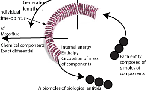﻿ Four laws of biology | Engenetics: The science of species

# The four laws of biology

## In the allowed set is at least one path such that mass is surrendered, and such that a further entity possessing the required set, and satisfying these four laws, results.

##### Explanation

G  O    T  O    M  A  X  I  M  S

dU = Mdt = δQ - dH; M > 0
Biology is “the study of those systems that can replace their internal energy” (See explanation of terms and variables).

pdt + mdt = dh + du = δq; m > 0
Ecology is “the study of the processes systems use to replace their internal energy” (See explanation of terms and variables).

The Gibbs-Duhem equation governs all biological energy:
m̅μ = dS = dU + dH - Σi μi(dvi - dmi)
The Euler equation governs all biological activity:
μ = dS = (∂S/∂U)V,Ni dU + (∂S/∂V)U,Ni dV + Σi (∂S/∂ui)U,V,{Nj≠i} dui + Σi (∂S/∂vi)U,V,{Nj≠i} dvi.

All biological populations are subject to the three constraints of:

(a) constant propagation, ;
(b) constant size, ;
(c) constant equivalence, .MAXIM 1: The maxim of dissipation
[Darwin's theory of competition]
M = nm̅;   ∫dm < 0;   ∇ • M → 0

MAXIM 2: The maxim of number
∇ • H = H/n =

MAXIM 3: The maxim of succession
[Darwin's theory of evolution]
∇ x M = ∂/∂t - ∂n/∂t

MAXIM 4: The maxim of apportionment
∇ x H = ∂/∂t - ∂n/∂t - ∂V/∂t